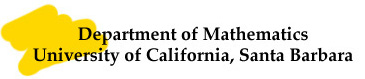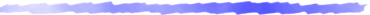### Publications

• Adiabatic limits, the non-multiplicativity of signature and Leray spectral sequence, Journal of A. M. S. vol 4 (1991), pp 256-321. pdf file

• Geometric invariants and their adiabatic limits. 145--156, Proc. Sympos. Pure Math., 54, Part 2, Amer. Math. Soc., Providence, RI, 1993. pdf file

• Eta invariants and determinant lines, J. Math. Phys., 35(1994), 5155-5194. with Dan Freed pdf file

• Negative Ricci curvature and isometry group, Duke Math J., 76(1994), 59-73. with Zhongmin Shen and Guofang Wei pdf file

• Finite part of the spectrum and isospectrality, Contemp. Math 173(1994), pp. 99-107 with Guofang Wei pdf file

• The Atiyah-Patodi-Singer index theorem: a proof via embedding, C. R. Acad. Sci, Paris, Series I, 319(1994), pp 1293-1297. with Weiping Zhang pdf file

• Invariants eta et droites determinants, C. R. Acad. Sci., Paris, Series I, 320(1995), pp 585-591. with Dan Freed pdf file

• Circle bundles and the Kreck-Stolz invariant, Trans. A. M. S., 347(1995), pp 3587-3593. with Weiping Zhang pdf file

• A Toponogov type comparison estimate for the Ricci curvature, Math. Ann. 303(1995), 297-306. with Guofang Wei pdf file

• Higher spectral flows, Math. Res. Letters 3(1996), 93-102. with Weiping Zhang pdf file

• Smoothing Riemannian metrics with Ricci curvature bounds, Manu. Math. 90(1996), 46-61. with Guofang Wei and Rugang Ye pdf file

• Splitting of the family index, Comm. Math. Phys. 182(1996), pp 303-317. with Weiping Zhang pdf file

• Higher spectral flows, J. Funct. Anal. 157(1998), pp 432-469. with Weiping Zhang pdf file

• Analytic torsion and R-torsion for manifolds with boundary, Asian J. Math. 4(2000), pp695-714. with Hao Fang pdf file

• Integral Pinching Theorems, Manu. Math. 101(2000), pp143-152. with Peter Petersen and Guofang Wei pdf file

• Real embeddings and the Atiyah-Patodi-Singer index theorem for Dirac operators, Asian J. Math. 4(2000), pp775-794. with Weiping Zhang pdf file

• APS boundary conditions, eta invariants and adiabatic limits, Trans. AMS, 354(2002), pp107-122. pdf file

• A heat kernel lower bound for integral Ricci curvature, Michigan J. Math. 52(2004), pp61-69. with Guofang Wei pdf file

• A Positive Mass Theorem for Spaces with Asymptotic SUSY Compactification, Comm. Math. Phys., 244(2004), 335-345. pdf file

• On the asymptotic expansion of Bergman kernel, C. R. Acad. Sci, Paris, Series I 339(2004), 193-198. with Kefeng Liu and Xiaonan Ma pdf file

• A Note on Positive Energy Theorem for Spaces with Asymptotic SUSY Compactification, Journal of Mathematical Physics, 46(2005), 042505-1 -- -7. pdf file

• Eta invariant and conformal cobordism, Annals of Global Analysis and Geometry, 27(2005), 333-340. pdf file

• On the Stability of Riemannian Manifold with Parallel Spinors, Invent. Math. 161(2005), 151-176. with Xiaodong Wang and Guofang Wei pdf file

• On the asymptotic expansion of Bergman kernel, Journal of Differential Geometry 72(2006), 1-41. with Kefeng Liu and Xiaonan Ma pdf file

• Eta invariants for manifold with boundary, Analysis, Geometry and Topology of Elliptic Operators, World Scientific and Imperial College Press, 2006, 141-172 pdf file

• An index theorem for Toeplitz operators on odd dimensional manifolds with boundary, J. Funct. Anal. 238 (2006), no. 1, 1--26. with Weiping Zhang pdf file

• Hitchin-Thorpe Inequality for Noncompact Einstein $4$-Manifolds, Advance in Math. 214 (2007) , no. 2, 551-570. with Guofang Wei pdf file

• Mass under the Ricci flow, Comm. Math. Phys. 274 (2007), 65-80. with Li Ma pdf file

• On the variational stability of Kahler-Einstein metrics, Comm. Anal. Geom. 15 (2007), 669-693. with Xiaodong Wang and Guofang Wei pdf file

• Stability of Einstein metrics and spin structures, Proceedings of the 4th International Congress of Chinese Mathematicians (2007), Vol. II, 59-72. pdf file

• $L^2$-cohomology of Spaces of Non-isolated Conical Singularity and Non-multiplicativity of signature, Progress in Mathematics (2009), 271 Birkh\"auser, 1-24. with Jeff Cheeger pdf file

• Adiabatic limit, Bismut-Freed connection and real analytic torsion form, Journal f\"ur die reine und angewandte Mathematik (Crelle's Journal), 2010. with Weiping Zhang pdf file

• An Introduction to L2 Cohomology, to appear in  Topology of Stratified Spaces, MSRI Publications, vol. 58,  2010. pdf file

• Adiabatic limit, heat kernels and analytic torsions, Progress in Mathematics, Vol 297 Birhauser， 233-298。 with Richard Melrose pdf file

• A remark on weighted Bergman kernel on orbifolds, Math Research Letters, 19 (2012), no. 01, 1–6. with K. Liu and X. Ma pdf file

• Eta invariants for even dimensional manifolds, Fifth International Congress of Chinese Mathematicians. Part 1, 2, 51–60, AMS/IP Stud. Adv. Math., 51, pt. 1, 2, Amer. Math. Soc., Providence, RI, 2012. pdf file

• Eta Invariant and Holonomy: the even dimensional case, Adv. In Math. 279(2015), 291-306. with W. Zhang pdf file

• Comparison between two analytic torsions on orbifolds, Math Z.(2017) 285:1269–1282 with J. Yu pdf file

• Local Sobolev constant estimate for integral Ricci curvature bounds, Adv. in Math. 325 (2018), 1-33.  with G. Wei and Z. Zhang pdf file

• Neumann isoperimetric constant estimate for convex domains, Proc. AMS 146 (2018), no. 8, 3509-3514. with G. Wei and Z. Zhang pdf file

• Perelman’s $\lambda$-functional for Manifolds with Conical Singularities, J. Geom. Anal., with C. Wang pdf file

• Fundamental gap estimate for convex domains on sphere -- the case n=2,  Comm. Anal. Geom., with S. Seto, G. Wei pdf file

### Books and Lecture Notes

• Metric and Differential Geometry, the Jeff Cheeger Anniversary Volume. Progress in Mathematics, Vol 297 Birhauser. Co-edited with X. Rong

• From probability to geometry (I), Volume in honor of the 60th Birthday of Jean-Michel Bismut， Asterique, vol. 327, 1-424. Co-edited with Remi Leandre, Xiaonan Ma and Weiping Zhang.

• From probability to geometry (II), Volume in honor of the 60th Birthday of Jean-Michel Bismut， Asterique, vol. 328, 1-393. Co-edited with Remi Leandre, Xiaonan Ma and Weiping Zhang.

• Lectures On Dirac Operators And Index Theory pdf file

• Comaprison Geometry for Ricci Curvature, with Guofang Wei pdf file